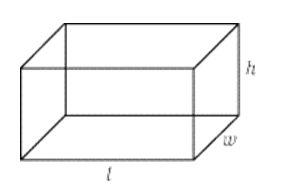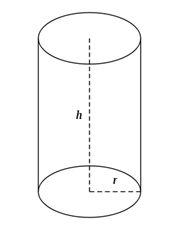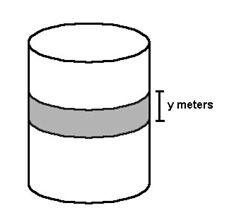# GMAT Solid Geometry - Rectangular Solids and Cylinders

Rectangular Solid

Learn the concepts behind volume and surface area before you start solving GMAT Solid geometry problems. All solid geometry problems come down to this - length, breadth and height. For data sufficiency questions, look out for values of l, b and h. if any of them are missing then it would be easy to eliminate answer choices.6 rectangular faces constitute a rectangular solid

The formulas you need to remember for a rectangular solid are

Volume = Length (l) x Width (w) x Height (h)

Surface Area = (2 x Length x Width) + (2 x Length x Height) + (2 x Width x Height)

"If length = width = height, that means that the rectangular solid is, in fact, a cube."

Terminologies

Vertex: Wow! quite a confusing word? Not really

Vertex = Corner

a) Vertex is the number of corners in a figure

The rectangular solid above has 8 vertices (plural of vertex), can you identify them?

b) Edge is the number of lines that you see in a diagram.

The edge is simply the lines you see in the diagram above: the line where two surfaces meet.

Cylinder

Questions that has cylinder in it are much easier. You have to focus on only two values - the radius(r) and the height(h).

If you don’t know the radius, anything that enables you to determine the radius, such as the diameter (radius = diameter / 2) or the circumference (radius = circumference / 2pi) will suffice.Regarding cylinders, the formulas you need to know are

Volume = area of the base circle x height = pi x (radius)2 x height

Surface Area = (2 x pi x (radius)2 )+ (pi x (diameter) x height)

Q) Let’s try a problem: A cylindrical water tank has a stripe painted around its circumference, as shown in the figure provided. What is the surface area of this stripe?

(1) y = 0.7
(2) The height of the tank is 2 meters.To find the surface area of the stripe, you need to know the circumference of the cylinder, but there is not data in the question that gives you the radius or diameter to let you find the circumference.  Hence the answer should be that neither statement together is sufficient.

Author :

Grockit, an online test prep game, is the smartest way to study for your test. It's adaptive, fun and finds the right teacher for you. Grockit’s analytic capabilities and adaptive technology identifies students' strengths and weaknesses, focusing the student's study time.  Students can practice in adaptive solo games, play social learning games with peers, and work with experts that match their specific needs.1. Complete GMAT RC Questions in less than 1 minute and 50 seconds
3. Take Notes Effectively
4. Collect and Interpret Facts
5. Speed up Summary Creation
6. Remember Information
7. Question the Author

12. Learn to Answer GMAT organization of passage Question
13. Learn to identify the style/tone or attitude of the author

Mastering GMAT Critical ReasoningAfter you read F1GMAT’s Mastering GMAT Critical Reasoning Guide, you will learn:

How to overcome flawed thinking in GMAT Critical Reasoning?

How to spot Inconsistencies in Arguments

How to eliminate out of scope answer choices using Necessary and Sufficient Conditions

How to Paraphrase GMAT Critical Reasoning Question

How to Answer Assumption Question Type

How to Answer Conclusion Question Type

How to Answer Inference Question Type

How to Answer Strengthen Question Type

How to Answer Weaken Question Type

How to Answer bold-faced and Summary Question Types

How to Answer Parallel Reasoning Questions

How to Answer the Fill in the Blanks Question

Get F1GMAT's Newsletters (Best in the Industry)

• Ranking Analysis
• Post-MBA Salary Trends
• Post-MBA Job Function & Industry Analysis
• Post-MBA City Review
• MBA Application Essay Tips
• School Specific Essay Tips
• GMAT Preparation Tips